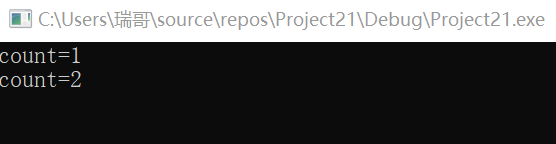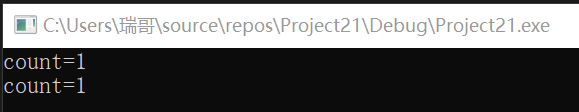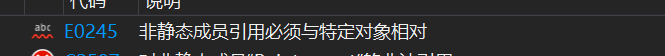# 第三次作业

### 类的静态成员

#include<iostream>
using namespace std;

class Client {
private:
static int count;
int id;
public:
Client(int id=0):id(id){
count++;
}
void showcount()
{
cout << "count=" << count << endl;
}
};
int Client::count = 0;

int main()
{
Client p(121);
p.showcount();
Client a(232);
a.showcount();
return 0;
}### 静态函数成员

1.静态成员函数是类中的特殊的成员函数
2.因为静态成员函数不属于某个对象，为该类的所有对象共享，所以静态成员函数没有this指针．
3.静态成员函数可以通过类名直接访问
4.静态成员函数可以通过对象访问
5.静态成员函数只能直接访问静态成员变量（函数），而不能直接访问普通成员变量（函数）

class A{
public:
static void f(A a);
private:
int x;
};
void A::f(A a){
cout<<x;//对x的引用是错误的
cout<<a.x;//正确
}


#include<iostream>
using namespace std;
class Point {
public:
Point(int x = 0, int y = 0) :x(x), y(y) {
count++;
}
Point(Point &p) {
x = p.x;
y = p.y;
count++;
}
~Point() {
count--;
}
int getX() { return x; }
int getY() { return y; }
static void showCount() {
cout << "count=" << count << endl;
}
private:
int x, y;
static int count;
};
int Point::count = 0;

int main()
{
Point a(4, 5);
cout << "Point A:" << a.getX() << "," << a.getY() << endl;
Point::showCount();

Point b(a);
cout << "Point B:" << b.getX() << "," << b.getY() << endl;
Point::showCount();

return 0;
}posted @ 2019-09-25 22:28  七岁就很酷  阅读(145)  评论(0编辑  收藏  举报